### Home > CALC > Chapter 4 > Lesson 4.2.4 > Problem4-87

4-87.
1. Evaluate the following integrals. Homework Help ✎

1.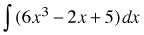2.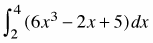3.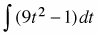4.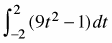5.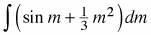6.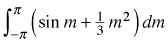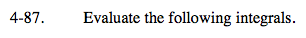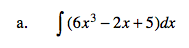'Undo' the Power Rule.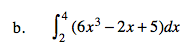Use your answer from part (a) to compute the integralon the interval from 2 to 4.

358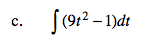See the hint from part (a).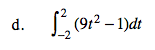Use your answer from part (c) to compute the integral on the interval from −2 to 2.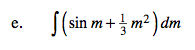Remember that the integral of sin(x) is −cos(x) + C.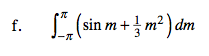Use your answer from part (e) to compute the integral on the interval from −π to π

$\frac{2\pi ^3}{9}$